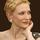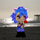## Social Question# Brain Teaser: Why does this trick work?

Asked by LostInParadise (28574) September 5th, 2009

Start with any set of numbers. To be specific I will use the following numbers: 2 3 5 7 10.

Choose any two numbers and replace them with the number you get by adding their product and sum. For example, if you choose 2 and 3, replace them with (2*3)+(2+3)=11. Now choose two other numbers on the list and keep repeating the process until there is one number left. Your final number will be 6335.

Obviously this works because the order the numbers are chosen makes no difference. But why should that be? This problem is a fairly simple demonstration of an important mathematical technique.

Observing members: 0Composing members: 0Let’s try it.

Starting:
4 6 20 800 38 72

First iteration, replacing 4 and 38:
(4 * 38) + (4 + 38) = 152 + 42 = 194

Result (new number in italics):
194 6 20 800 72

Second iteration, replacing 6 and 800:
(6 * 800) + (6 + 800) = 4800 + 806 = 5606

Result (new numbers in italics):
194 5606 20 72

Is there something you didn’t tell us?

I chose a even number of starting numbers, six. (You said choose any set.)
I chose a large number. (You didn’t specify a range.)
I chose all even numbers. (You didn’t specify odds and evens.)

I have two left. How am I going to get to one, and how am I going to get an odd number out of all evens?

Jeruba (51640)“Great Answer” (1) Flag as…I should have been clearer in my explanation. What I should have said is that for any set of numbers the answer that you get will be the same for that set of numbers regardless of the order in which they are chosen. I did not mean to imply that the answer would be the same regardless of the set of numbers chosen. I apologize if that is the impression that I gave.

LostInParadise (28574)“Great Answer” (0) Flag as…I wouldn’t call it a trick at all, then. It’s just a relationship. There’s another way of expressing those component relationships, and somehow it has to do with the properties of addition and the fact that multiplication is repeat addition (so that the summing is the same as multiplying each of the factors by one more), and that’s as far as my English-major brain is going to be able to go with it.

Jeruba (51640)“Great Answer” (1) Flag as…@Jeruba I admire you. There is no way I can explain those, muchless even do it.

brinibear (1388)“Great Answer” (0) Flag as…@Jeruba , When I said trick I did not mean actual magical conjuring. This is mathematics after all. You are correct is saying that the key is finding another way of expressing the formula xy + x + y.

As a hint to those who may be intimidated by this problem,
xy + x + y can almost, but not quite, be factored. The attempt at factoring, along with the necessary correction leads to a general formula when terms are combined.

LostInParadise (28574)“Great Answer” (0) Flag as…I have the feeling I am not going to get any more takers on this, so here is the solution.

xy + x + y = (x+1)(y+1) – 1
Now see how this works.
Starting with 2 and 3 we get (2+1)(3+1) – 1, which replaces 2 and 3
Now let’s combine this with 5.
We get:
[(2+1)(3+1)-1 +1]((5+1) – 1 = (2+1)(3+1)(5+1) – 1

Given k terms, the final answer is (n1 + 1)(n2+1)...(nk+1) – 1

As @Jeruba suggested, the answer comes from the properties of arithemtic. Since it does not matter what order the multiplication occurs in, the answer does not depend on the order that the terms are combined. In the example given, the answer is (2+1)(3+1)(5+1)(7+1)(10+1) -1 =6335

I don’t know if anybody is going to read this, but here is a quick lesson on the general principle being used.
Let f(x) = xy = x + y
Then f(x) + 1 = (x+1)(y+1)
Now let T(x) = x+1, and let g(x) = x*y
We have for the general case:
T(f(x) = g(T(x),T(y))

Why is this of any use? Let me show you a way of looking at this that even English majors can understand. Suppose I want to know the value of f(x,y), but I want to have someone else do it. I find an expert on the calculation, but she speaks some foreign language. I then hire a translator. Think of T(x) as translation. The translation of f is g and of x and y are T(x) and T(y). This will work provided that g(T(x),T(y)) represents a translation of the answer, that it is equal to T(f(x,y). The translator can translate in answer given as f(x,y). In other words, the translation process sets up a kind of parallel world. If the translation is easier to work with or provides additional information then it can be very useful.

For example, the use of logarithms.
log(x*y) = log(x) + log(y). Here T(x) = log(x), f(x,y)=(x*y) and g(x,y) = x + y. This can prove useful because addition is easier to work with than multiplication. Mathematical transforms, of which there are many, like Fourier and Laplace transforms, use the same idea.

Consider any mathematical model, like the determination of fuel cost for a trip based on distance, gas mileage and gas cost. The use of any mathematical model is based on translating certain real world measurements into numerical values and then using the model to mimic the way these measured values work together to compute some other real world value. If the model and measurements are accurate, it is this same translation idea.

LostInParadise (28574)“Great Answer” (0) Flag as…